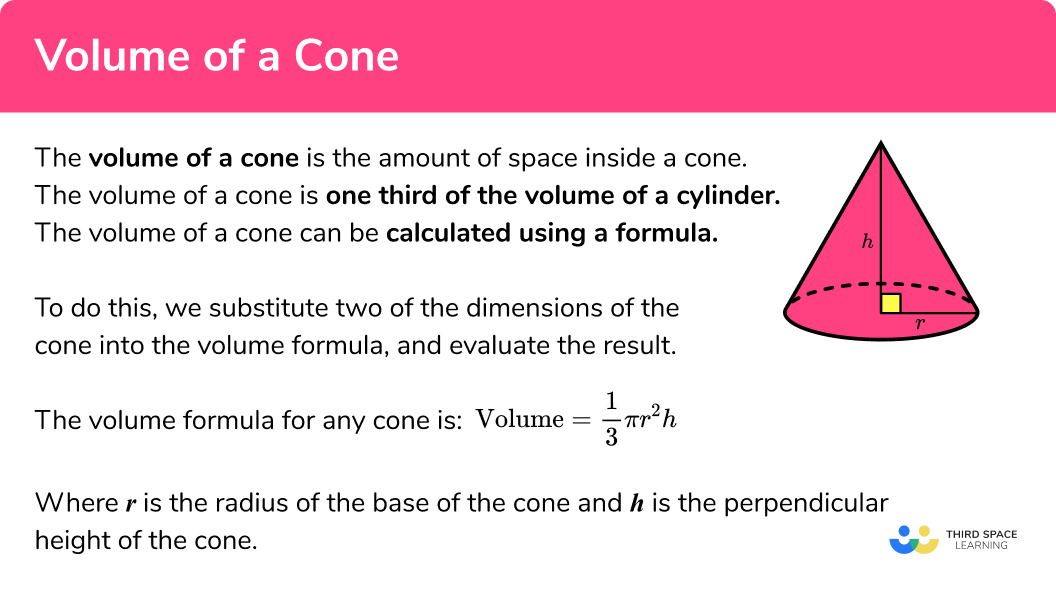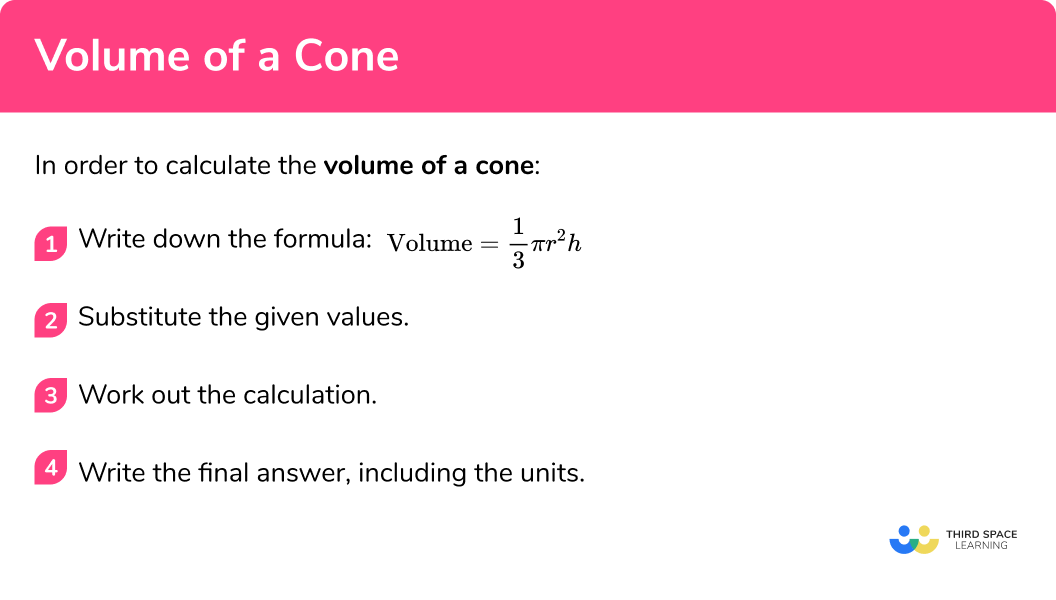# Volume Of A Cone

Here we will learn about the volume of a cone, including how to calculate the volume of a cone given its radius and perpendicular height and how to calculate a missing length within a cone, given its volume.

There are also cone worksheets based on Edexcel, AQA and OCR exam questions, along with further guidance on where to go next if you’re still stuck.

## What is the volume of a cone?

The volume of a cone is the amount of space inside a cone.

The volume of a cone is one third of the volume of a cylinder.

The volume of a cone can be calculated using a formula.

To do this, we substitute two of the dimensions of the cone into the volume formula and evaluate the result.

The volume formula for any cone is

\text{Volume}=\frac{1}{3} \pi r^2 h

where r is the radius of the base of the cone and h is the perpendicular height of the cone.

### What is the volume of a cone?### Example

Find the volume of this cone where the radius of the base of the cone is 5cm and the perpendicular height of the cone is 8cm .

We need to find the area of the base of a cone using A=\pi r^{2} (the area of a circle).

We then multiply it by the vertical height h , and find one third of the answer.

$\text{Volume}=\frac{1}{3} \pi r^2 h\\ = \frac{1}{3} \times \pi \times 5^2 \times 8\\ =\frac{200}{3}\pi\\ =209.4 \ cm^3 \ \text{(1 dp)}$

### Types of cones

A cone is a three dimensional object with a circular base that tapers to a point (a vertex). This vertex is also known as the apex.

If the apex is directly above the centre of the base, the cone is referred to as a right circular cone. If the apex is not above the centre of the base, this is referred to as an oblique cone.

Note: The slant height of the cone l is not needed to calculate the volume of a cone, but it is used to calculate the surface area of a cone.

Step-by-step guide: Surface area of a cone (coming soon)

The volume of a cone stays the same if the apex moves parallel to the base but the surface area of the cone changes. This is because the slant heights are not equal for an oblique cone.

## How to calculate the volume of a cone

In order to calculate the volume of a cone:

1. Write down the formula, \text{volume}=\frac{1}{3} \pi r^2 h .
2. Substitute the given values.
3. Work out the calculation.
4. Write the final answer, including the units.

### How to calculate the volume of a cone### Related lessons on cones

Volume of a cone is part of our series of lessons to support revision on cone. You may find it helpful to start with the main cone lesson for a summary of what to expect, or use the step by step guides below for further detail on individual topics. Other lessons in this series include:

## Volume of a cone examples

### Example 1: integer dimensions

Find the volume of the cone with radius 4cm and perpendicular height 7cm .

1. Write down the formula, \text{volume}=\frac{1}{3} \pi r^2 h .

The formula for the volume of a cone is V=\frac{1}{3} \pi r^2 h .

2Substitute the given values.

We need to substitute the value of the radius r and the perpendicular height h into the formula.

V= \frac{1}{3}\times\pi\times{4^2}\times{7}

3Work out the calculation.

Use a calculator to work out the volume.

V=\frac{112}{3}\pi = 117.286…

4Write the final answer, including the units.

Check what form the final answer needs to be. Here we are asked to give the answer to 3 significant figures.

V=117.286…=117 cm^3 (3sf)

The volume of the cone is 117cm^3 (3sf) .

### Example 2: decimal dimensions

Find the volume of the cone with radius 2.1cm and perpendicular height 4.3cm .

Write down the formula, \text{volume}=\frac{1}{3} \pi r^2 h .

Substitute the given values.

Work out the calculation.

Write the final answer, including the units.

### Example 3: calculate the volume in terms of pi

Calculate the volume of a cone with radius 3cm and perpendicular height 5cm .

Write down the formula, \text{volume}=\frac{1}{3} \pi r^2 h .

Substitute the given values.

Work out the calculation.

Write the final answer, including the units.

### Example 4: calculate the volume given the diameter

Find the volume of the cone with the diameter of 4cm and perpendicular height 6cm .

Write down the formula, \text{volume}=\frac{1}{3} \pi r^2 h .

Substitute the given values.

Work out the calculation.

Write the final answer, including the units.

### Example 5: calculate a length given the volume

A cone has a volume of 500cm^3 and a radius of 5cm . Calculate the perpendicular height of the cone, correct to 1 decimal place.

Write down the formula, \text{volume}=\frac{1}{3} \pi r^2 h .

Substitute the given values.

Work out the calculation.

Write the final answer, including the units.

### Example 6: calculate the radius given the volume

A cone has a volume of 800cm^3 and a perpendicular height of 15cm . Calculate the radius of the cone. Give your answer to 2 decimal places.

Write down the formula, \text{volume}=\frac{1}{3} \pi r^2 h .

Substitute the given values.

Work out the calculation.

Write the final answer, including the units.

### Common misconceptions

• Using the correct formula

There are several formulas that can be used, so we need to match the correct formula to the correct context.

• Rounding

It is important to not round the answer until the end of the calculation. This will mean your final answer is accurate.

• Using the radius or the diameter

It is a common error to mix up radius and diameter. Remember the radius is half of the diameter.

• Make sure you have the correct units
• For area we use square units such as cm^2 .
• For volume we use cube units such as cm^3 .

### Practice volume of a cone questions

1. Find the volume of a cone of radius 7.3cm and perpendicular height 9.7cm . Give your answer to 3 significant figures.541 cm^3542 cm^3719 cm^3718 cm^3Substitute the values of r and h into the formula.

V=\frac{1}{3}\pi r^2 h

V=\frac{1}{3}\times \pi \times 7.3^2\times 9.7

V=541.310…

V=541 \ cm^3 \ \text{(3sf)}

2. Find the volume of a cone of radius 8.6cm and perpendicular height 7.9cm . Write your answer to 3 significant figures.562 cm^3563 cm^3611 cm^3612 cm^3Substitute the values of r and h into the formula.

V=\frac{1}{3}\pi r^2 h

V=\frac{1}{3}\times \pi \times 8.6^2 \times 7.9

V=611.860…

V=612 \ cm^3 \ \text{(3sf)}

3. Calculate the volume of a cone with the radius 6cm and the perpendicular height 7cm . Write your answer in terms of \pi .42\pi cm^384\pi cm^3126\pi cm^3252\pi cm^3We are finding the volume of a cone so we substitute the values of r and h into the formula.

V=\frac{1}{3}\pi r^2 h\\
V=\frac{1}{3}\times \pi \times 6^2 \times 7\\
V=84\pi\\
V=84\pi \ cm^{3}

4. A cone has a diameter of 8cm and a perpendicular height of 6cm . Calculate the volume of the cone in terms of \pi .12\pi cm^332\pi cm^348\pi cm^396\pi cm^3Calculate the value of the radius by dividing the diameter by 2 , then substitute the values of r and h into the formula and solve.

r=8 \div 2= 4cm

V=\frac{1}{3} \pi r^2 h

V=\frac{1}{3}\times \pi \times 4^2 \times 6

V=32\pi

V=32\pi \ cm^3

5. A cone has a volume of 400cm^3 . The radius of the base is equal to 6cm . Calculate the perpendicular height of the cone to 1 decimal place.

3.5 cm8.2 cm10.6 cm11.1 cmSubstitute the volume and the radius into the volume formula and then rearrange to find the perpendicular height, h .

V=\frac{1}{3} \pi r^2 h

400=\frac{1}{3}\times \pi \times 6^2 \times h

1200=\pi \times 36 \times h

\frac{1200}{\pi \times 36}=h

h=10.610…

h=10.6 \ cm \ \text{(1dp)}

6. The volume of a cone is 700 cm^3 . The perpendicular height of the cone is 8cm . Calculate the radius of the cone to 2 decimal places.

5.40 cm5.72 cm9.14 cm9.28 cmSubstitute the values of the volume and the perpendicular height into the formula, and rearrange to find the radius r .

V=\frac{1}{3} \pi r^2 h

700=\frac{1}{3}\times \pi \times r^2 \times 8

2100=\pi \times r^2 \times 8

\frac{1200}{\pi \times 8}=r^2

r=\sqrt{\frac{2100}{\pi \times 8}}

r=9.1409…

r=9.14 \ cm \ \text{(to 2 dp)}

### Volume of a cone GCSE questions

\text{Volume of a cone}=\frac{1}{3} \pi r^2 h

1. Below is a cone with radius 4.1 cm and perpendicular height 5.8 cm .Calculate the volume of the cone.

(2 marks)

V=\frac{1}{3} \times \pi \times 4.1^2 \times 5.8

(1)

102.099…=102

(1)

2. Use the information in the diagram below to calculate the volume of the 3D shape.(2 marks)

V=\frac{1}{3} \times \pi \times 8^2 \times 12

(1)

256\pi

(1)

3. A container is a cone of radius 30 cm and perpendicular height 50 cm .Water fills the container at a rate of 3000 cm^3 per minute.

How long does it take to fill the container?

(4 marks)

\frac{1}{3} \times \pi \times 30^2 \times 50

(1)

= 47 123.8898…

(1)

47 123.8898… \div 3000=15.707…

(1)

15.707… = 16 minutes

(1)

## Learning checklist

You have now learned how to:

• Calculate the volume of a cone

## Still stuck?

Prepare your KS4 students for maths GCSEs success with Third Space Learning. Weekly online one to one GCSE maths revision lessons delivered by expert maths tutors.

Find out more about our GCSE maths tuition programme.